# Variables & Data Types – PHP Basics

Variables are the storage of different types of information. You cannot start with a number while declaring a variable and it starts with ‘\$’ sign followed by variable name (for ex. \$code). It can only contain underscore and alpha numeric characters. ‘\$code’ and ‘\$CODE’ are two different variables here because its case sensitive.

Declaring Variables :

Here to declare a variable ‘\$’ sign is used.

```<!DOCTYPE html>
<html>
<body>

<?php
\$text = "Code Projects";
\$a = 0;
\$b = 1;

echo \$text;
echo "<br>";
echo \$a;
echo "<br>";
echo \$b;
?>

</body>
</html>```

Output :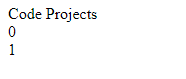Output Variables :

```<!DOCTYPE html>
<html>
<body>

<?php
\$text = "code-projects.org";
echo "Projects, Tutorial and More on :- \$text";
?>

</body>
</html>```

Output :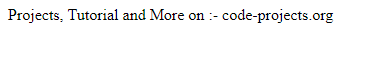To Display Sum of Variables:

```<!DOCTYPE html>
<html>
<body>

<?php
\$a = 10;
\$b = 20;
\$c = 30;
echo \$a + \$b + \$c;
?>

</body>
</html>```

Output :Variable Scopes

Local, Global, Static are the three different Variable Scopes.

Local & Global Scope :

Variable created within a function and only access within that function is Local Scope.
Variable created outside a function and only access outside a function is Global Scope.

Local:

```<!DOCTYPE html>
<html>
<body>

<?php
function code() {
\$a = 1; // LOCAL SCOPE
echo "<p>Variable a inside function is: \$a</p>";
}
code();

// Using variable 'a' outside the function will generate ERROR
echo "<p>Variable a outside function is: \$a</p>";
?>

</body>
</html>```

Output :Global :

```<!DOCTYPE html>
<html>
<body>

<?php
\$a = 1; // GLOBAL SCOPE

function code() {
// Using variable 'a' inside this function will generate ERROR
echo "<p>Variable a inside function is: \$a</p>";
}
code();

echo "<p>Variable a outside function is: \$a</p>";
?>

</body>
</html>```

Output :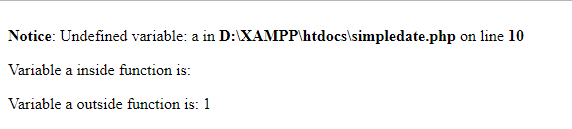The Global Keyword :

To access global variable from within a function, Global Keyword is used. For this you must use Global keyword before variables inside the function.
All global variables are stored in an array called \$GLOBALS[index]. here Index holds name of variable.

```<!DOCTYPE html>
<html>
<body>

<?php
\$a = 10;
\$b = 20;

function code() {
\$GLOBALS['b'] = \$GLOBALS['a'] + \$GLOBALS['b'];
}

code();
echo \$b;
?>

</body>
</html>```

Output :Static Keyword :

All of its variables are removed when a function is executed. Sometimes we need a Local variable and don’t want it to be removed for our future purpose. For this, use Static keyword when you create a variable. then only each time when you run  a function, information will be still in that variable contained from last time when function was called.

```<!DOCTYPE html>
<html>
<body>

<?php
function code() {
static \$a = 10;
echo \$a;
\$a++;
}

code();
echo "<br>";
code();
echo "<br>";
code();
?>

</body>
</html>```

Output :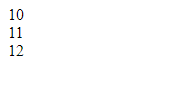DATA TYPES :

Types are :- String, Integer, Float, Boolean, Array, Object, NULL, Resource

String :

Arrangement of Characters is String. It can be any text inside a single Quotation Mark(‘ ‘) or Double Quotation Mark (” “).

```<!DOCTYPE html>
<html>
<body>

<?php
\$a = "Hello Everyone. Thanks For Choosing Code Projects";
\$b = 'Hello Everyone. Thanks For Choosing Code Projects';

echo \$a;
echo "<br>";
echo \$b;
?>

</body>
</html>```

Output :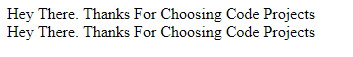Integer :

Its a non-decimal number between -2,147,483,648 and 2,147,483,647. Integer must contain at least a digit, it can be either negative and positive and it must not have any decimal points. In these three formats : Decimal (10-based), HexaDecimal (16-based – prefixed with 0x) or Octal (8-based – prefixed with 0), integer can be described.

```<!DOCTYPE html>
<html>
<body>

<?php
\$a = 012345;
var_dump(\$a);
?>

</body>
</html>```

Output: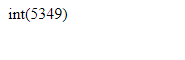Float :

Its a number in exponential form or a number containing Decimal point.
float ex. \$b = 1.11;   //\$b is float

```<!DOCTYPE html>
<html>
<body>

<?php
\$b = 20.0123;
var_dump(\$b);
?>

</body>
</html>```

Output :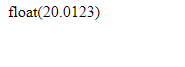Boolean :

It produce 2 possbile states. They are TRUE or FALSE. It is mostly used in conditional testing.

\$a = true;
\$b = false;

Array :

An array stores many value in a single variable.

\$name = array(“john”, “johnny”,”harry”);   //here ‘\$name’ is an array

```<!DOCTYPE html>
<html>
<body>

<?php
\$langs = array("PHP","C#","PYTHON","JAVASCRIPT");
var_dump(\$langs);
?>

</body>
</html>```

Output :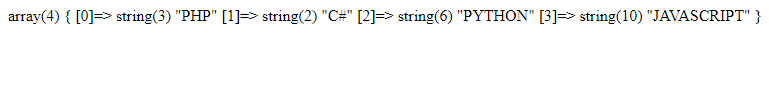Object :

It stores data & information on how to process that data. Here in PHP an object must be explicitly created.

At first create a class of object. and for this you must use class keyword.
A class is a structure that involves properties and methods.

```<!DOCTYPE html>
<html>
<body>

<?php
class Codes {
function Codes() {
\$this->lang = "PHP";
}
}
// Create an object
\$prp = new Codes();

// Show Object Properties
echo \$prp->lang;
?>

</body>
</html>```

Output :NULL Value :

It can only have one value. i.e NULL. A variable that has no value assigned to it is null value data type. Variable is automatically Null when it is created without a Value.

```<!DOCTYPE html>
<html>
<body>

<?php
\$a = "Hello There. Thanks For Choosing Code Projects";
\$a = null;
var_dump(\$a);
?>

</body>
</html>```

Output :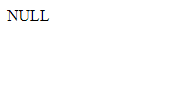Resource :

It’s not an actual data type. It’s the storing of a reference to functions and resources external to PHP.

Database call is an example of using resource.

While performing operations like connecting database or opening an external file, resource data will return. When we connect database with the required configuration details like,

```//connection

The function will return resource type data to be stored into ‘\$conn’ variable.

We can retrieve needed list of data items from the database with reference to resource created.